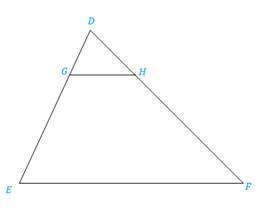Chapter 5.3, Problem 22E### Elementary Geometry for College St...

6th Edition
Daniel C. Alexander + 1 other
ISBN: 9781285195698

#### Solutions

Chapter
Section### Elementary Geometry for College St...

6th Edition
Daniel C. Alexander + 1 other
ISBN: 9781285195698
Textbook Problem
1 views

# In Exercises 17 to 24, complete each proof.Given: D G D E = D H D F Prove: ∠ D G H ≅ ∠ EPROOF Statements Reasons 1. ?2. ∠ D ≅ ∠ D 3. Δ D G H ∼ Δ D E F 4. ? 1. ?2. ?3. ?4. ?

To determine

To prove:

The statement DGHE if the proportions are given DGDE=DHDF.

Explanation

Definition:

SAS:

If an angle of one triangle is congruent to an angle of a second triangle and the pairs of sides including the angles are proportional (in length), then the triangles are similar.

CASTC:

Corresponding angles of similar triangles are congruent.

Description:

Given that DGDE=DHDF.

The given figure is shown below.

Figure

From the given figure, it is observed that DGDE=DHDF are the pairs of corresponding sides which are proportional and also noticed that the common point of both triangles is D.

Hence, by identity DD.

The above mentioned SAS definition, the two triangles DGH and DEF are similar since an angle of one triangle is congruent to an angle of a second triangle and the pairs of sides including the angles are proportional (in length)

### Still sussing out bartleby?

Check out a sample textbook solution.

See a sample solution

#### The Solution to Your Study Problems

Bartleby provides explanations to thousands of textbook problems written by our experts, many with advanced degrees!

Get Started

#### Convert the expressions in Exercises 8596 radical form. 94(1x)7/3

Finite Mathematics and Applied Calculus (MindTap Course List)

#### Write the following numbers in word form. 2.48

Contemporary Mathematics for Business & Consumers

#### It does not exist.

Study Guide for Stewart's Multivariable Calculus, 8th